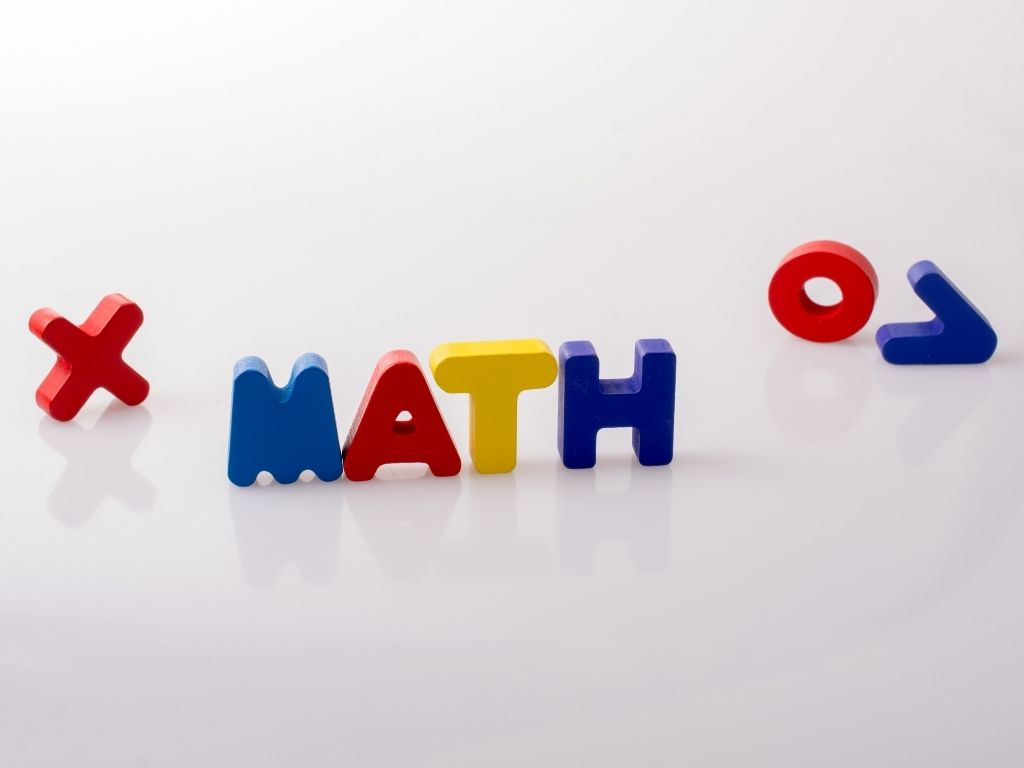## Polynomials Class 10th

Introduction A polynomial word is made up of two Greek terms “poly” which means many and “nominal” which means terms So, polynomial means many terms. Polynomials are the combined expression of variables, constants, and exponents (only whole numbers) with arithmetic expressions like addition, subtraction, multiplication, and division (division by only constant terms). Polynomial can have …